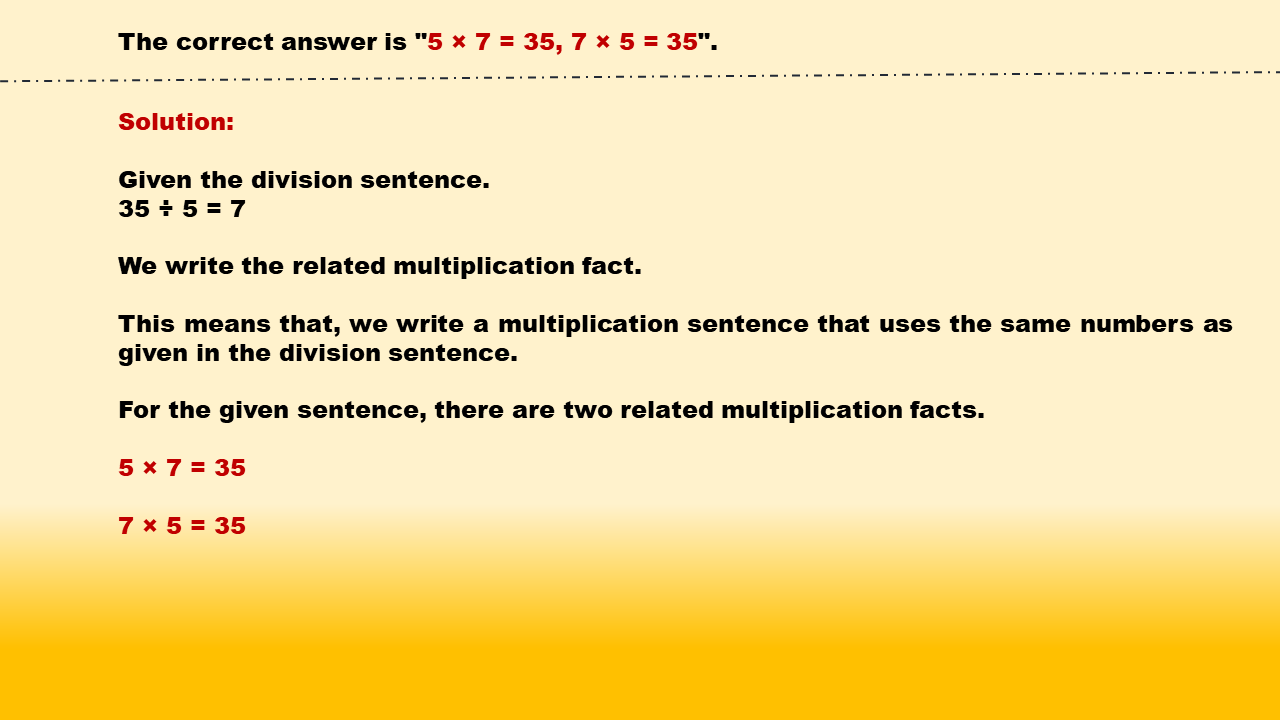1
visibility

Identify related multiplication facts for 35 ÷ 5 = 7.

• 5 × 6 = 30, 6 × 5 = 30

• 5 × 4 = 20, 4 × 5 = 20

• 5 × 7 = 35, 7 × 5 = 35

• 5 × 8 = 40, 8 × 5 = 40